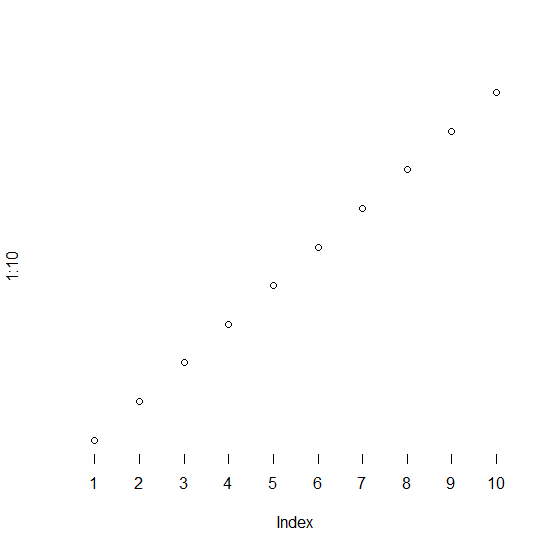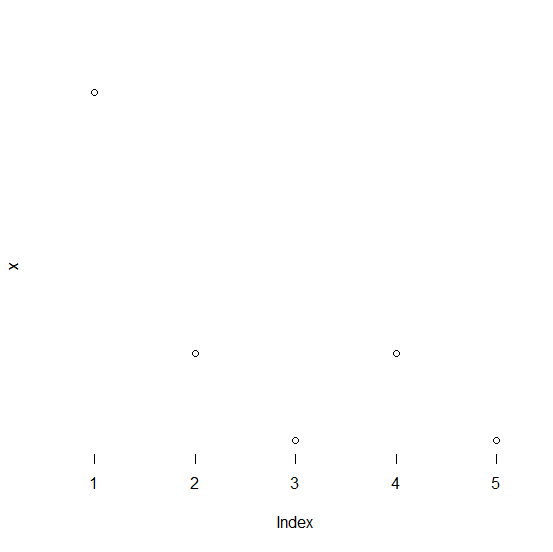# How to create a plot in base R with tick marks but excluding axes lines?

To create a plot with tick marks but without axes lines, we first need to create the plot without axes and then add the tick marks. This can be done with the help of plot function and axis function in base R. The axis function will help us to decide where do we need the tick marks and the ticks.

## Example1

> plot(1:10,axes=FALSE)
> axis(1,c(1:10),col=NA,col.ticks=1)

## Output## Example2

Live Demo

> x<-rpois(5,2)
> x

## Output

 5 2 1 2 1

## Example

> plot(x,axes=FALSE)
> axis(1,c(1:5),col=NA,col.ticks=1)

## Output